# Key Parameters When Using Dynamic Light Scattering

An important parameter of colloidal systems is their particle size. However, the particle size can be obtained by several techniques. So which one must be selected? Comparing stated dimensions from one technique with those from another can be complicated and should be employed with caution: distributions in terms of number, volume, or scattering intensity usually produce extremely varying results — in spite of expressing precisely the same physical characteristics of a sample.

In this article, a titanium dioxide sample has been used as an example for highlighting the differences between the three distributions.

## What is a Distribution?

Several things in nature have characteristics that demonstrate variations. An explanation of the probability of encountering a specific value of a variable is called a distribution. For instance, an age distribution of a group of people might reveal the number of members of that group in every age category. This type of distribution would precisely be signified as an “age distribution by number.” Three types of distributions are generally encountered when particle size distributions are important.

The number distribution demonstrates the number of particles in the various size bins. The volume distribution demonstrates the total volume of particles in various size bins. The intensity distribution gives the amount of light scattered by the particles in the different size bins. Are these three distributions different? The answer is yes.

Consider a simple example of a distribution containing only two particles, particle species one with size a and particle species two of size b. If there are Na particles with the size a and Nb particles with size b, then in terms of number, their relative contributions compared to the other particle are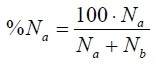(1)

where %Na is the relative number of the particle with size a. Supposing that the particles are spherical, the particle volume is equivalent to the size to the third power. Consequently, the relative contribution from a in terms of volume is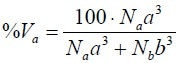(2)

where %Va is the relative volume of the particle with size a. Lastly, for small, isotropic particles, the scattering intensity from a spherical particle is equivalent to the size to the sixth power. Consequently, the relative contribution from a in terms of intensity is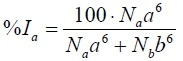(3)

where %Ia is the relative amount of intensity of the particle with size a.

In the case of real-life distributions, the situation can be modeled in a manner similar to the simplified two-particle model used above as an example.

Practically, this usually means that the intensity distributions highlight the larger particles in the distribution; in contrast, the number distributions highlight the smaller particles in the distribution. Yet, it is vital to observe that both are simply different representations of the same physical reality of a distribution of various sizes.

The above isotropic assumption holds true for particles that are considerably smaller than the wavelength of the illuminating light, usually 632.8 nm in the Zetasizer Nano. A complete description of the highly complex scattering behavior involves the application of Mie scattering. This theory elucidates the amount of light scattered by a particle of any size when the entire optical properties (absorption and refractive index) are known. The intensity scattered based on Mie theory is not simply equivalent to the size to the sixth power; rather, it is altered by a size-dependent Mie function M(a). The generalized form of Equation 3 then becomes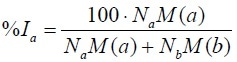(4)

The direct data acquired from a light scattering experiment is in accordance with these %intensity values for the intensity distribution.

## Size Distribution by Intensity

Figure 1 illustrates the intensity size distribution from TiO2 particles in water. The histogram (steps) rather than the frequency curve (smooth) representation is revealed for the purpose of indicating the discrete nature of the regularization algorithm. The mean size obtained by intensity is 87 nm with a standard deviation of 32 nm. Table 1 lists the obtained key size parameters.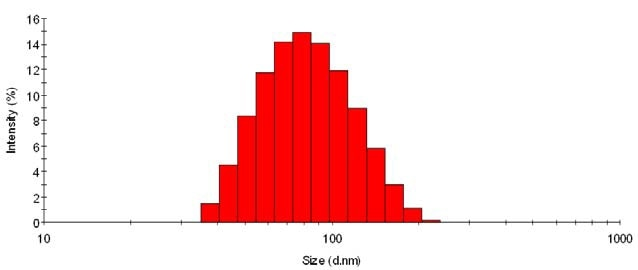Figure 1. Size distribution by intensity, %intensity versus size in nm.

Table 1. Mean size and standard deviation obtained from a sample of TiO2 in water. The values depend on the distribution chosen.

Mean [nm] Width [nm]
Intensity 87 32
Volume 65 25
Number 50 14

## Size Distribution by Volume

Figure 2 illustrates the volume size distribution from the sample TiO2 particles in water. In this article, the mechanism as explained in Equation 4 is used for back-calculating the distribution that describes the measured intensity as observed in Figure 1. The shape of the distribution is quite different, and seems to be biased toward smaller sizes. The mean size obtained by volume is now 65 nm with a standard deviation of 25 nm.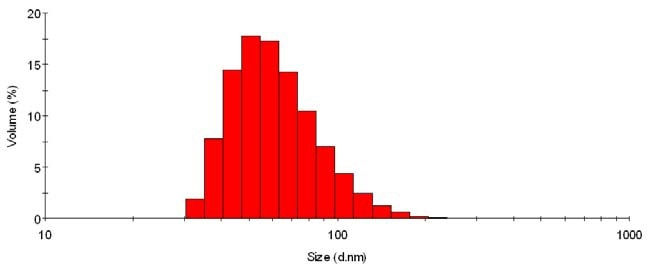Figure 2. Size distribution by volume, %volume versus size in nm.

## Size Distribution by Number

The intensity size distribution from the sample TiO2 may also be transformed into a number distribution, as illustrated in Figure 3. The shape of the distribution is evidently different and absolutely improves the magnitude of the smaller size bands. The mean size obtained from this representation is 50 nm, with a standard deviation of 14 nm — these values are by number.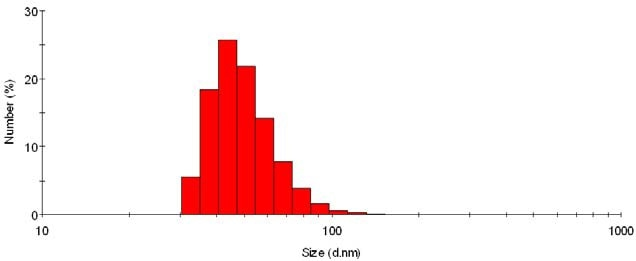Figure 3. Size distribution by number, %number versus size in nm.

## Which is the Correct Size?

All three are correct is the simplest answer to this question. If it is evidently stated how the size was obtained and what distribution representation was considered, any of the size distribution offers useful data related to the sample.

For realistic applications, it is advantageous to note that comparison of several samples must always be performed with the same distribution analysis. Put differently, two different samples, measured by light scattering, should only be compared by analyzing the same distribution — preferably the intensity as those data are closest to what is actually measured, and do not involve any assumptions about the Mie scattering function.

For small (less than 300 nm) particles, and those for which the parameters for the Mie function are illustrious, reliable volume and number distributions can be compared and overlaid, with the condition that the “raw” data quality is excellent enough, so that the distribution conversion intensity → volume → number is valid.

## Comparison with Other Methods

The detection mechanism of technologies must be considered while comparing light scattering data with other sizing methods. For instance, an RI or UV detector coupled with chromatography detects the concentration of material in the eluted phase. Consequently, the distribution acquired from such a measurement is efficiently a concentration, or alternatively a volume or — for the case of a homogeneous population of material of common density — a mass distribution. Thus, the volume distribution from light scattering would compare best with RI or UV chromatography data.

In (electron) microscopy, particles are presented as numbers in each size bin, that is, the obtained average size is one which is closest corresponding to the number distribution from light scattering.This information has been sourced, reviewed and adapted from materials provided by Micromeritics Instrument Corporation.

## Citations

• APA

Micromeritics Instrument Corporation. (2019, December 09). Key Parameters When Using Dynamic Light Scattering. AZoM. Retrieved on October 20, 2020 from https://www.azom.com/article.aspx?ArticleID=16772.

• MLA

Micromeritics Instrument Corporation. "Key Parameters When Using Dynamic Light Scattering". AZoM. 20 October 2020. <https://www.azom.com/article.aspx?ArticleID=16772>.

• Chicago

Micromeritics Instrument Corporation. "Key Parameters When Using Dynamic Light Scattering". AZoM. https://www.azom.com/article.aspx?ArticleID=16772. (accessed October 20, 2020).

• Harvard

Micromeritics Instrument Corporation. 2019. Key Parameters When Using Dynamic Light Scattering. AZoM, viewed 20 October 2020, https://www.azom.com/article.aspx?ArticleID=16772.